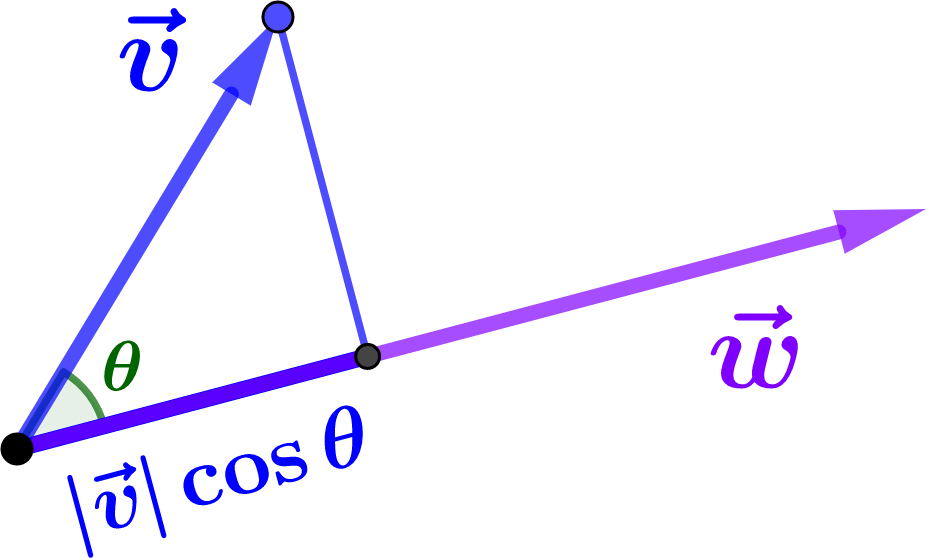## Section1.7The Dot Product

Before you read this section, you should take a few minutes to jot down everything you know about the dot product. Many students feel that if they know one representation for a concept, that is sufficient. As you will discover throughout this book, the professional scientist's ability to solve problems often comes from the ability to play off several different representations against each other.

The geometric definition of the dot product is given by:

\begin{equation} \vv\cdot\ww = {|\vv| |\ww| \cos\theta}\tag{1.7.1} \end{equation}

where $$\theta$$ is the angle between $$\vv$$ and $$\ww\text{.}$$ Now we notice something interesting: If $$\vv$$ is the vector along the hypotenuse of the triangle in Figure 1.7.1, then $$|\vv|\cos\theta$$ is the projection of $$\vv$$ onto the direction of $$\ww\text{,}$$ the other vector. Similarly, $$|\ww| \cos\theta$$ is the projection of $$\ww$$ onto the direction $$\vv\text{,}$$ so the dot product is a symmetrized version of projection.Figure 1.7.1. A projection can be found using the geometric definition of the dot product.

More precisely, in Figure 1.7.1, the length of the heavy line segment represents the component of $$\vv$$ in the $$\ww$$ direction. If we regard this component as the definition of the dot product of $$\vv$$ with the unit vector $$\ww\over|\ww|\text{,}$$ then multiplying through by $$|\ww|$$ and using some triangle trigonometry leads immediately to Equation (1.7.1) for the dot product of $$\vv$$ and $$\ww\text{.}$$ (Projecting $$\ww$$ onto $$\vv$$ instead leads to the same result.)

Thus, the dot product is a rescaled projection. It is this rescaling that makes it symmetric; it doesn't matter which vector is which.

Since the angle between a vector and itself is zero, an immediate consequence of this formula is that the dot product of a vector with itself gives the square of its magnitude, that is

\begin{equation} \vv\cdot\vv = |\vv|^2 .\tag{1.7.2} \end{equation}

In particular, taking the “square” of any unit vector yields $$1\text{,}$$ for example

\begin{equation} \xhat\cdot\xhat = 1 .\tag{1.7.3} \end{equation}

Furthermore, since $$\cos{\pi\over2}=0\text{,}$$ it also follows immediately from the geometric definition that two (nonzero) vectors are orthogonal if and only if their dot product vanishes, that is  1

\begin{equation} \vv\perp\ww \Longleftrightarrow \vv\cdot\ww = 0 .\tag{1.7.4} \end{equation}

Because the dot product is distributive (i.e. you can "FOIL" the dot product over a sum of vectors),  2  the geometric formula Equation (1.7.1) can be used to express the dot product in terms of vector components. For example, if $$\vv=v_x\,\xhat+v_y\,\yhat$$ and $$\ww=w_x\,\xhat+w_y\,\yhat\text{,}$$ then

\begin{align} \vv\cdot\ww \amp = (v_x\,\xhat+v_y\,\yhat)\cdot(w_x\,\xhat+w_y\,\yhat) \notag\\ \amp = v_x w_x \,\xhat\cdot\xhat + v_y w_y \,\yhat\cdot\yhat + v_x w_y \,\xhat\cdot\yhat + v_y w_x \,\yhat\cdot\xhat\notag\\ \amp = v_x w_x + v_y w_y\tag{1.7.5} \end{align}

which is often given as the algebraic definition of the dot product in rectangular coordinates in two dimensions. A similar formula holds in three dimensions.

A special case is the dot product of a vector with itself, which reduces to the Pythagorean theorem when written out in terms of components, for example

\begin{equation} \vv\cdot\vv = |\vv|^2 = v_x^2 + v_y^2 .\tag{1.7.6} \end{equation}

In the above computation we simply “multiplied out” the original expression in terms of components, then used the multiplication table

\begin{gather*} \xhat\cdot\xhat = 1 = \yhat\cdot\yhat ,\\ \xhat\cdot\yhat = 0 = \yhat\cdot\xhat . \end{gather*}

Using the multiplication table of basis vectors under the dot product in this way is a useful strategy which you are encouraged to practice.

Returning to our earlier example of motion at 3 mph to the east and 4 mph to the north, we have $$\uu=3\,\xhat+4\,\yhat\text{,}$$ so that

\begin{gather*} |\uu|^2 = \uu\cdot\uu = 3^2 + 4^2 = 5^2 \end{gather*}

and the overall speed is $$|\uu|=5~\hbox{mph}$$ as expected.

### Activity1.7.1.Combining the algebraic and geometric definitions of the dot product.

Find the angle between a diagonal of a cube and one of its edges

Solution.

Consider the unit cube in the first octant. The vertices that we care about are at $$(0,0,0), (1,0,0), (1,1,1)\text{.}$$ The length of the vector from the origin along the diagonal of the cube is $$\sqrt{3}$$ and the length of the vector along the edge is 1. By setting the dot product of these two vectors, calculated using the geometric formula Equation (1.7.1) and also the algebraic formula, equal to each other, we can solve for the cosine of the angle that we want.

\begin{align*} \xhat\cdot(\xhat+\yhat+\zhat) \amp = \vert \xhat\vert \, \vert (\xhat+\yhat+\zhat)\vert \, \cos\gamma\\ 1 \amp = \sqrt{3}\cos\gamma\\ \cos\gamma \amp = \frac{1}{\sqrt{3}} \end{align*}

Since we know from the diagram that the angle we are looking for is less than $$\frac{\pi}{2}\text{,}$$ choose the angle for the arccosine in the first quadrant.

Since the zero vector $$\zero$$ also satisfies this condition, it is, by definition, orthogonal to every other vector!
There is some fine print here: we must show that the dot product is, in fact, linear in its arguments, so that it distributes over addition as claimed. This property can be established geometrically; see our online article 3 .
mathdl.maa.org/mathDL/4/?pa=content&sa=viewDocument&nodeId=1156